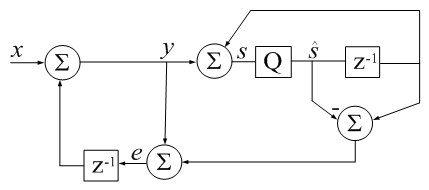# Accumulator Error Feedback

(Difference between revisions)
 Revision as of 20:28, 29 January 2018 (edit)← Previous diff Revision as of 20:42, 29 January 2018 (edit) (undo)Next diff → Line 17: Line 17: % % % Example: % Example: + % clear all; clc % csumv=0; rsumv=0; % csumv=0; rsumv=0; % n = 100e6; % n = 100e6; Line 41: Line 42: === sorting === === sorting === - Sorting is not integral above because the commented Example + In practice, input sorting can sometimes achieve more accurate summation. - (inspired by Higham) would then display false positive results.
+ Compensated sum accuracy is quite data dependent. - In practice, input sorting + Substituting a sine wave of randomized frequency, instead of a random number sequence input, - should begin the csum() function to achieve the most accurate summation: + can make compensated summation fail to produce more accurate results than a simple sum. -
+                                                               Sorting became integral to later algorithms, such as those from Knuth and Priest.
-                                                            function s_hat = csum(x)                                                                                                       +                                                               But the very same accuracy dependence on input data prevails.
-                                                            s_hat=0; e=0;                                                                                                                  +
-                                                            [~, idx] = sort(abs(x),'descend');                                                                                             +
-                                                            x = x(idx);                                                                                                                    +
-                                                            for i=1:numel(x)                                                                                                               +
-                                                            s_hat_old = s_hat;                                                                                                             +
-                                                            y = x(i) + e;                                                                                                                  +
-                                                            s_hat = s_hat_old + y;                                                                                                         +
-                                                            e = y - (s_hat - s_hat_old);  %calculate parentheses first                                                                     +
-                                                            end                                                                                                                            +
-                                                            return                                                                                                                         +
-
+ - Even in complete absence of sorting, csum() can be more accurate than conventional summation by orders of magnitude. + === links === === links ===

## Revision as of 20:42, 29 January 2018csum() in Digital Signal Processing terms: z-1 is a unit delay,
Q is a 64-bit floating-point quantizer. Algebra represents neither a sequence of instructions or algorithm. It is only meant to remind that an imperfect accumulator introduces noise into a series.
qi represents error due to quantization (additive by definition).
```function s_hat = csum(x)
% CSUM Sum of elements using a compensated summation algorithm.
%
% This Matlab code implements Kahan's compensated
% summation algorithm (1964) which often takes about twice as long,
% but produces more accurate sums when the number of
% elements is large. -David Gleich
%
% Also see SUM.
%
% Example:
% clear all; clc
% csumv=0;  rsumv=0;
% n = 100e6;
% t = ones(n,1);
% while csumv <= rsumv
%    v = randn(n,1);
%
%    rsumv = abs((t'*v - t'*v(end:-1:1))/sum(v));
%    disp(['rsumv = ' num2str(rsumv,'%1.16f')]);
%
%    csumv = abs((csum(v) - csum(v(end:-1:1)))/sum(v));
%    disp(['csumv = ' num2str(csumv,'%1.16e')]);
% end

s_hat=0; e=0;
for i=1:numel(x)
s_hat_old = s_hat;
y = x(i) + e;
s_hat = s_hat_old + y;
e = y - (s_hat - s_hat_old);
end
return
```

### sorting

In practice, input sorting can sometimes achieve more accurate summation. Compensated sum accuracy is quite data dependent. Substituting a sine wave of randomized frequency, instead of a random number sequence input, can make compensated summation fail to produce more accurate results than a simple sum. Sorting became integral to later algorithms, such as those from Knuth and Priest. But the very same accuracy dependence on input data prevails.